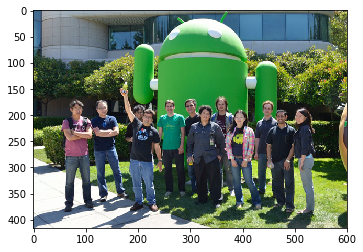# Lecture Notes: Basic Image Processing¶

Before jumping to build powerful and intelligent models for visual recognition it is always important to look at some pixels. Looking at images and pixels and transforming them in various ways gives us often valuable intuitions on how to find things about images, and how to build the algorithms behind intelligent visual recognition systems. We will be using pytorch's Tensors to manipulate images as tensors, and the pillow (PIL) image processing library.

In :
import torch
import numpy as np
from PIL import Image
import matplotlib.pyplot as plt
import torchvision.transforms as transforms
%matplotlib inline

# pytorch provides a function to convert PIL images to tensors.
pil2tensor = transforms.ToTensor()
tensor2pil = transforms.ToPILImage()

# Read the image from file. Assuming it is in the same directory.
rgb_image = pil2tensor(pil_image)

# Plot the image here using matplotlib.
def plot_image(tensor):
plt.figure()
# imshow needs a numpy array with the channel dimension
# as the the last dimension so we have to transpose things.
plt.imshow(tensor.numpy().transpose(1, 2, 0))
plt.show()

plot_image(rgb_image)

# Show the image tensor type and tensor size here.
print('Image type: ' + str(rgb_image.type()))
print('Image size: ' + str(rgb_image.size()))Image type: torch.FloatTensor
Image size: torch.Size([3, 416, 600])


The rgb_image variable contains a torch.FloatTensor of size channels x height x width corresponding to the dimensions of the image. Each entry is a floating-point number between 0 and 1.

## 1. Image channels¶

We can slice the image into each R, G, and B channels and show them separately:

In :
from io import BytesIO
import IPython.display

r_image = rgb_image
g_image = rgb_image
b_image = rgb_image

def show_grayscale_image(tensor):
# IPython.display can only show images from a file.
# So we mock up an in-memory file to show it.
# IPython.display needs a numpy array with channels first.
# and it also has to be uint8 with values between 0 and 255.
f = BytesIO()
a = np.uint8(tensor.mul(255).numpy())
Image.fromarray(a).save(f, 'png')
IPython.display.display(IPython.display.Image(data = f.getvalue()))

# Cat concatenates tensors along a given dimension, we choose width here (1), instead of height (0).
show_grayscale_image(torch.cat((r_image, g_image, b_image), 1))